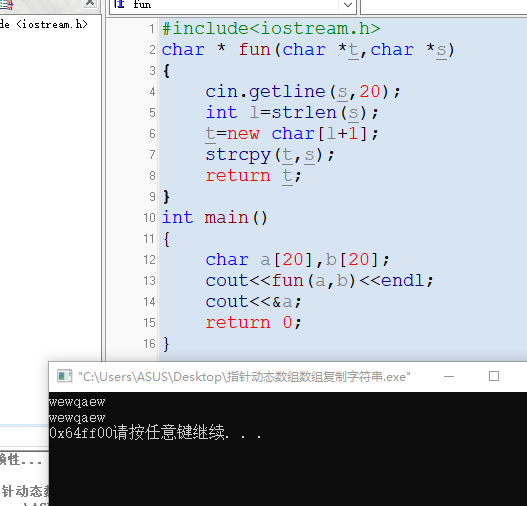• **为何输出成员brand是乱码“屯”？** #include #include using namespace std; int main() { struct CandyBar { char brand; float weight; int calorie; }; CandyBar *test=new CandyBar; test.brand ...
• #include<stdio.h> #include<stdlib.h> int main() ...Error 9 error C2059: syntax error : ')' c:\users\thinkpad\documents\c语言挑战题\pocketnum\...小白不懂二维动态数组怎么定义，求提携。。。c语言
• 有个动态数组输出的问题，请大家帮忙看看，老师要求可以在任意位置插入或删除动态数组的值。这里还没实现任意性，只是先试试插入指定位置的值。不过最后输出的时候，在数组输出完成后跟了“-842150451”。找不到原因...
• 【算法代码】 #include <bits/stdc++.h> using namespace std; vector<int> v; int main() { int x; while(cin>>x) { v.push_back(x); } sort(v.begin(),v.end());...&l
【算法代码】

#include <bits/stdc++.h>
using namespace std;

vector<int> v;

int main() {
int x;
while(cin>>x) {
v.push_back(x);
}

sort(v.begin(),v.end());

//	for(vector<int>::iterator it=v.begin(); it!=v.end(); it++) {
//		cout<<*it<<" ";
//	}

for(int i=0;i<v.size();i++){
cout<<v[i]<<" ";
}

return 0;
}



展开全文数据结构 STL vector
• new这个关键字 1、系统分配一段内存空间 2、调用构造函数 3、返回指针 我自己写的时候，将字符串复制到动态数组的时候就出了问题，我想输出这个字符串，因为我们平时只要输出数组名就可以了，所以我打算输出实参，...
#include<iostream.h>
char * fun(char *t,char *s)
{
cin.getline(s,20);
int l=strlen(s);
t=new char[l+1];
strcpy(t,s);
return t;
}
int main()
{
char a,b;
cout<<fun(a,b)<<endl;
cout<<&a;
return 0;
}
关于动态数组理解起来不是很难，在c++中是用的new定义，delete释放   c是malloc定义，free释放，使用动态数组可以有效利用空间
int size=20;
int *p=new int[size]；
二维的话就要
int size=20；
int (*p)=new int [size]；
new这个关键字
1、系统分配一段内存空间
2、调用构造函数
3、返回指针
我自己写的时候，将字符串复制到动态数组的时候就出了问题，我想输出这个字符串，因为我们平时只要输出数组名就可以了，所以我打算输出实参，就导致了乱码，在老师的帮助下我理解到了这个实参a并没有输入，是不确定的，就会乱码，可以直接用cout<<函数完成返回值的输出。
附上运行截图展开全文指针 字符串 c++
• malloc动态内存分配练习 从键盘输入成绩并打印在屏幕上，人数未知 */ #include #include int main() { int num=0; printf("请输入学生人数：\n"); scanf("%d",&num); int *mark; mark = (int *)malloc(num ...malloc 内存分配
• 分别使用数组静态和动态初始化方式初始化一个大小为10的整型数组输出。 import java.util.Scanner; public class java_array_1 { //1)、使用数组静态初始化和动态方式初始化一个大小为10的整型数组输出。 ...
分别使用数组静态和动态初始化方式初始化一个大小为10的整型数组并输出。
import java.util.Scanner;
public class java_array_1 {
//1)、使用数组静态初始化和动态方式初始化一个大小为10的整型数组并输出。

public static void main(String[] args) {
int[] arry1={10,12,23,34,56,78,78};//数组静态化，初始并且赋值
for(int i=0;i<arry1.length;i++){
System.out.println(arry1[i]);
}
int[] arry2= new int;//动态定义个数组并且赋值
Scanner imput = new Scanner(System.in);
for (int i = 0; i < 10; i++) {
System.out.println("请给第"+(i+1)+"数组赋值");
arry2[i]=imput.nextInt();
}
for (int i = 0; i < 10; i++) {//输出通过动态定义的数组的值
System.out.println(arry2[i]);
}
}

}


注意：数组的定义格式
1、int [] A =new int ;
int A[] = new int ;
这两种定义格式一样的
2、数组的初始化问题
int  [] A = {1,2,3,4,5,6};
int  [] A = new int []{1,2,3,4,5,6};
这两种格式都可以的。
3、常见数组错位的定义方式
   a、  int  [] A = new int {1,2,3,4,5,6};
new int 后面的括号必须为空

b、   int arr;
arr={1,2,3,4};
不可以分成两不走的格式；
正确的格式为：int  [] arr= new int {1,2,3,4};

c、   int[] score = new int;
score={23,34,56,67,78};//此方法错误
数组定义分配空间后，给数值赋值，必须一个个的赋值，score[i]这种形式才对。 而score为数组的地址，因此score={23,34,56,67,78}这个方法赋值错误。

d、   int[] score2;
score={23,45,67,78,90,56};
此方法错误。

总结：数组定义赋值两种方式
一种方式为     int  [] A = {1,2,3,4,5,,6};
int  [] A = new int []{1,2,3,4,5,6};

另一种方式为 int [] A =new int ;
int A[] = new int ;
定义后，然后给数组的每一个元素赋值A[i]



展开全文java
• ## 动态数组存储输出

千次阅读 2008-10-17 13:49:00
那么也许应该 先定义一个长度较大的数组 进行一次循环 找到数组存储值最大的下标值 求和数组判断是否是完数 然后再进行一次循环 将数组输出 这样做会非常麻烦 程序很繁琐 例如 Java code public ...
比如求1000内完数的问题 完数 即该数的所有因子之和等于它本身 如6=1+2+3 用程序写一个求完数的程序 只需进行2次循环 比求质数的要难一点 课本只要求找到完数 那么程序会很容易写出来 Java code

public class ws{
public static void main(String []args)
{
int sum=0;
for(int i=1;i<1000;i++)
{
for(int j=1;j<=i/2;j++)
{
if(i%j==0)
{
sum+=j;
}
}
if(sum==i)
{
System.out.println(i);
}
sum=0;
}
}
}

但是如果想打印出完数的具体内容呢？比如要显示“6=1+2+3” 很容易想到的方法就是把1，2，3存入数组中 但是数组的长度是多少呢？ 那么也许应该 先定义一个长度较大的数组 进行一次循环 找到数组存储值最大的下标值 求和数组判断是否是完数 然后再进行一次循环 将数组输出 这样做会非常麻烦 程序很繁琐 例如 Java code

public class ws{
public static void main(String[] args)
{
int a,i,j=0,k=0,l,s=0;
int[] e;
for(a=1;a<=1000;a++)
{
for(i=1;i<=a/2;i++)
{
if(a%i==0)
{
//        System.out.println(i+"是"+a+"的因数");
k++;
if(i>=a/2-1){
e=new int;
for(i=1;i<=a/2;i++)
{
if(a%i==0)
{
e[j]=i;
//System.out.println("e["+j+"]的值为"+e[j]);
j++;
}
//j=0;
}
for(l=0;l<=j;l++)
{
s+=e[l];
}
if (s==a)
{
System.out.println(a+"是个完数");
System.out.print(a+"=1");
for(l=1;l<=j-1;l++)
{
System.out.print("+"+e[l]);
}
System.out.println();
}
s=0;
j=0;
}
}
}
// System.out.println("除"+a+","+a+"的因数有"+k+"个");
k=0;
}
}
}

对此虽然实现了想要的结果 但程序不能令我们满意 有没有更好的办法呢？ 集合类中的接口为我们提供了方法 关键字：Vector;Enumeration Vector类提供了类似动态数组的功能 但是它只能对对象进行操作 不过我们可以利用简单的数据类型转换来运用Vector类 然后通过Enumeration接口来实现动态数组的读取操作 这样我们只需要一次循环就可以完成数组的存储 无需判断数组的最大有效下标了 Java code

import java.util.*;
public class Zxzjava3 {
public static void main(String []args)
{
final int total=1000;
int sum=0;
Vector v=new Vector();
for(int i=1;i<total;i++)
{

for(int j=1;j<=i/2;j++)
{
if(i%j==0)
{
v.addElement(new Integer(j));
}
}
Enumeration e=v.elements();
while(e.hasMoreElements())
{
Integer intObj=(Integer)e.nextElement();
//System.out.println(intObj);
sum+=intObj.intValue();
}
if (sum==i)
{
System.out.println(i+"是个完数");
Enumeration f=v.elements();
System.out.print(i+"="+"1");
while(f.hasMoreElements())
{
Integer intObj=(Integer)f.nextElement();
if(intObj.intValue()==1)
{
}
else
System.out.print("+"+intObj.intValue());

}
System.out.println();
}
v.clear();
sum=0;
}
}
}


展开全文存储 integer vector string class
• 输出这个动态数组，和其中的最大值。 【知识点：ArrayList类的使用，随机数的使用】 输入描述 无 输出描述 分两行分别输出这个动态数组，和其中的最大值。 输入样例 无 输出样例 生成的动态数组为: [43, 39, 20, 40,...java 算法
• Console.Write("请输入动态数组的行数："); int row = Convert.ToInt32(Console.ReadLine()); Console.Write("请输入动态数组的列数："); int col = Convert.ToInt32(Console.ReadLine()); int[,] arr = new ...
• *问题描述:动态数组实现输出全班平均成绩 *作者:小臣小仁 *完成日期:2017年11月2日 */ int main() { float score;//存在隐患 float aver,total=0; int num,i=0; printf("请输入学生的人数:"); scanf("%d",...
• 1、初始化时设置他的数组大小 string[] myarr = new string; myarr = "1"; myarr="第二个值";2、初始化时设置他的数组大小并赋值...3、可以动态改变数组大小的数组 List listArr = new List(); listArr.add
• 当一行数据满足筛选要求时,将该行数据写入到新的数组中,逐维增加,最后再将这个新的数组输出到表格. 动态数组和一静态数组定义不同 静态数组定义: dim arr 动态数组定义: dim arr() 重新定义动态数组: ...数据结构 大数据 数据库 数据分析 spring
• 静态数组初始化格式 数据类型[] 数组名 = {元素1,元素2,元素3}; 实例:int[] arr = {11,22,33}; 动态数组初始化格式 int[] arr = new int;...静态数组与动态数组的输入输出比较 package com.itheima.kuih...
• #include&lt;stdio.h&gt;#include&lt;stdlib.h&gt;...//输入二维数组的行数 scanf("%d",&amp;row); a=(int **)malloc(sizeof(int)*row); printf("Please e...
• 一、数组分为动态数组和静态数组： 1.动态数组： int[] arr = new int[长度]; 动态数组是由系统自动分配数值，其默认值为0，如果对自动分配的数值不满意，可以给它赋值： arr[i] = 数值； //输出数组中的元素： ...JAVA
• 从键盘读入n个整数，使用动态数组存储所读入的整数，并计算它们的和与平均值分别输出。要求尽可能使用函数实现程序代码。平均值为小数的只保留其整数部分。 样例输入: 5 3 4 0 0 2 样例输出: 9 1 样例输入: 7 3 2 7 ...算法 python java c++ c语言
• #include #include #include using namespace std; void mp(int *p, int n) { int len = n; ... //设置输出结束时间  cout 一共用了" 毫秒" ; return 0; }C++ 随机数
• 这是根据动态二维数组输出一个稀疏矩阵，根据我自己想输入的稀疏矩阵来输出对应的三元组
• 　算法训练 动态数组使用 ...从键盘读入n个整数，使用动态数组存储所读入的整数，并计算它们的和与平均值分别输出。要求尽可能使用函数实现程序代码。平均值为小数的只保留其整数部分。 样例输入:...
• C#中的数组是可以动态分配长度的,实现动态数组有以下几种途径,这里说的动态数组包括动态分配大小和动态增加数据. 先来看原始数组:  int m = 5;  int[] array = newint[m];  array = 2;  label1.Text = ...
• 运用动态内存分配函数malloc，创建一个整数类型（int）的动态数组，首先输入动态数组的元素个数，然后再依次输入每个元素，最后输入一个起始下标（默认从0开始），程序将依次输出从该下标开始直到动态数组中最后一个...c语言
• 定义一个数组（整型），要求实现数据的添加，数组内容的输出数组大小的动态输出2.写一个子类继承，对实现输出数组实现进行排序3.写一个子类继承，对实现输出数组进行反转 */class Array{private int [] data; ...
• 2017-04-30编一个程序，输入某班...班级人数不定，要求采用动态数组完成。//项目2-动态数组排序 #include #include<malloc.h>//数组打印函数 void Print(float *x, int N) { int i, j; for(i=0, j=1; i; i++, j++) {c语言 设计
• 从键盘读入n个整数，使用动态数组存储所读入的整数，并计算它们的和与平均值分别输出。要求尽可能使用函数实现程序代码。平均值为小数的只保留其整数部分。 样例输入: 5 3 4 0 0 2 样例输出: 9 1 样例输入: 7 3 2 7 ...java 算法...